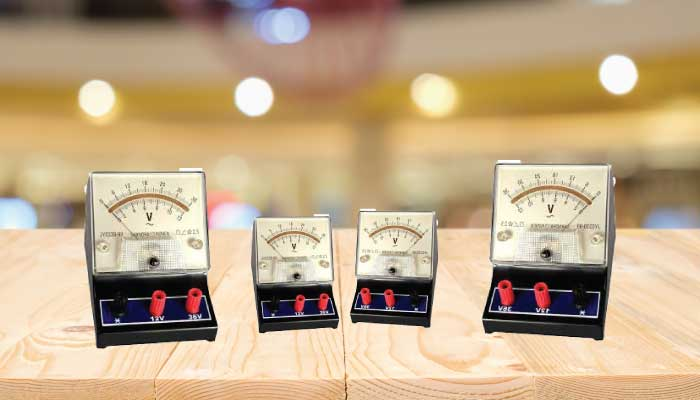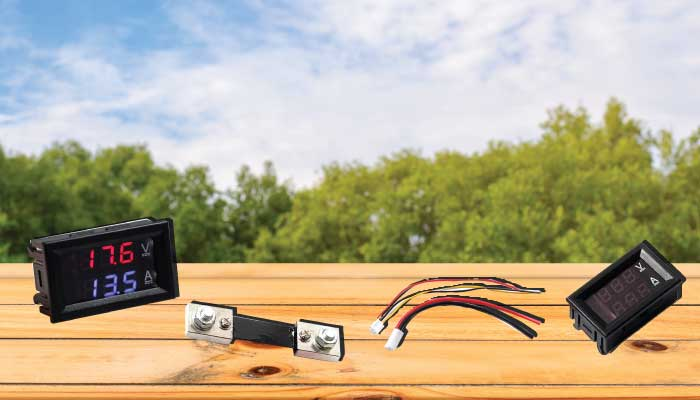# What is a voltmeter? how is its structure and operating principle?Similar to an ammeter or an Ohmmeter, a voltmeter is an important electrical device. Let’s find out about this device in the following article.

What is a voltmeter?

A voltmeter is an electronic device used to measure potential differences or voltages between two points in an electrical or electronic circuit. Potential difference units are measured in volts (V).Some types of voltmeter

The structure of a voltmeter

An analog voltmeter basically consists of a sensitive galvanometer (current meter) in series with high resistance. The internal resistance of the voltmeter must be high. On the other hand, it attracts a significant current and interrupts the circuit operation when the test is performed.

Voltmeters use an electronic display needle on the dial. The electronic voltmeter displays the numerical value of the voltage of the current on the electronic screen of the device.

Measurement range of Voltmeter

Laboratory voltages have a maximum range of 1000 – 3000 V. Most commercial meters have different ranges. For example 0-1 V, 0-10 V, 0-100 V and 0-1000 V. In computing, the standard laboratory voltmeter is suitable. Because the measured voltage is average. Usually, between 1 V and 15 V. Typically, an analog voltmeter is used to measure voltage from part of a volt to several thousand volts. In contrast, digital voltages are more accurate. They are used to measure very small voltages in laboratories and electronics.

History of the voltmeter

The basic principles of the voltmeter were invented by Danish physicist Hans Christian Oersted in 1820 when he discovered that an electric current in a wire produces a magnetic field around it. The first ammeter was invented by physicist André Ampère in 1820 to measure electric current.Hans Christian Oersted

Galvanometer

A galvanometer can also be modified to become an ammeter. It is used to measure larger currents. When this device is used to measure voltage, the galvanometer turns into a voltmeter connected parallel to the area it is measuring. Because the voltmeter has to be attached to a parallel circuit, it needs to be made in a way with high resistance.

In a circuit, the current will always follow the path of the smallest resistance. The potential difference then is in any part of the circuit. It is important to change that part as little as possible when making measurements.

How does the voltmeter work?

The general principle of the voltmeter is that it must be connected in parallel with the object to which you want to measure the voltage. This is used because the voltmeter has a high voltage value. So if the voltmeter is connected in series, the voltage will be almost zero. This means the circuit is open. In addition, in parallel circuits, it is known that the voltage at all points is the same. Thus the voltage difference between the voltmeter and source is almost the same.

In theory, for an ideal voltmeter, you should have resistance at infinity so that the current attracts zero; In this way, there is no power loss in the device.

But this is not feasible in practice because you cannot have a material with infinite resistance.

Practical application of the Voltmeter

Voltmeter allows to safely measure the voltage or voltage difference between two points of a circuit, and do not change the voltage of that circuit. The ability to measure voltage is very important to the voltmeter. Besides voltmeter also has other applications. For example, you want to turn on the fan but it doesn’t work. The fan may be damaged, the wall outlet may not be receiving power. The voltmeter can then be used to measure the voltage of the plug. If it is not around 120V, the plug may be damaged.

Another use of a voltmeter is to determine whether the battery is charged or discharged. When the car is not starting, you can measure the battery voltage with a voltmeter to see if there is a problem.

Common types of voltmeter

There are different types of voltmeter. Here are some common types:

• Iron voltmeter
• Inductive voltmeter
• Static voltmeter
• Permanent magnet voltmeter
• Rectifier voltmeter
• Digital voltmeterDigital voltmeterStatic voltmeter

Voltmeters designed to measure dangerous and high voltages have an extra layer of insulation between the test points and the user to prevent electric shock. There are also other types of voltmeter designed to measure low voltage in small objects, such as computer chips, with great accuracy.

The most common voltmeter is a handheld device that has a screen and two electronic cables. These cables are connected to two points in the circuit and the voltage level is displayed on the screen. This device not only measures the voltage. It can also measure resistance or amperage. It is called a multimeter.

Hope the information about Voltmeter has helped you to better understand this electric tool. See you in the next useful posts.

Source: Wikipedia

Beeteco.com is the e-commerce channel of Hao Phuong Corporation, specializing in distributing and trading industrial electrical equipment of many prestigious brands in the world.

Want more advice on the Voltmeter? => Call us immediately via Hotline: 1800 6547